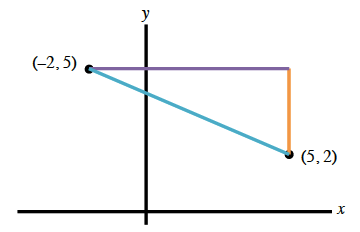### Home > INT3 > Chapter 2 > Lesson 2.2.2 > Problem2-69

2-69.

Consider the points $(-2,5)$ and $(5,2)$.

1. Calculate the distance between the points.  Give your answer both in radical form and as a decimal approximation.

Use the Pythagorean Theorem to find the distance.
$a^2+b^2=c^2$
$(7)^2+(3)^2=c^2$
$49+9=c^2$

$c=\sqrt{58}\approx7.62$ units1. What is the slope of the line that goes through the two points?

Plot the points on the graph using the eTool below. Find the distance and slope.
Click the link at right for the full version of the eTool: 2-69 HW eTool